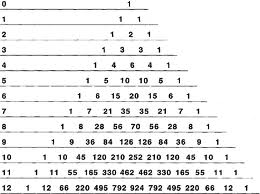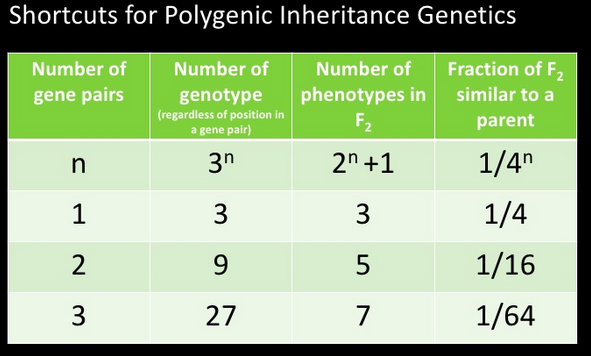# Polygenic inheritance

Sometimes in olympiads you can confront with tricky polygenic inheritance problems. As I noticed, all you need to know in order to successfully solve polygenic inheritance problems is to be acquainted with probabilities and understand Pascal’s triangle shown below:Pascal’s Triangle

The Pascal’s triangle represents the probability of “either-or” events, such as heads or tails in the tossing of a coin, and boy or girl in one-child births. The left-hand column shows the number of events, and is represented by n. For example, if a coin is tossed twice, n =2 and there is one chance of two heads, one chance of two tails, and two chances of one head and one tail (i.e., head and tail, tail and head). These probabilities are called binomial coefficients and the triangle is easily constructed because each coefficient is the sum of the two immediately above it, to its right and left. For any number of events, the largest binomial coefficient occurs with n/2 events. For example, in a family of eight children, the total possible combination of four boys and four girls is 70. With a gene-for-gene relationship that has twelve pairs of genes, the maximum number of locks and keys occurs when each lock and key has n/2 (i.e. 12/2 = 6) genes. From the triangle, it will be seen that there are 924 possible 6-gene locks and keys.